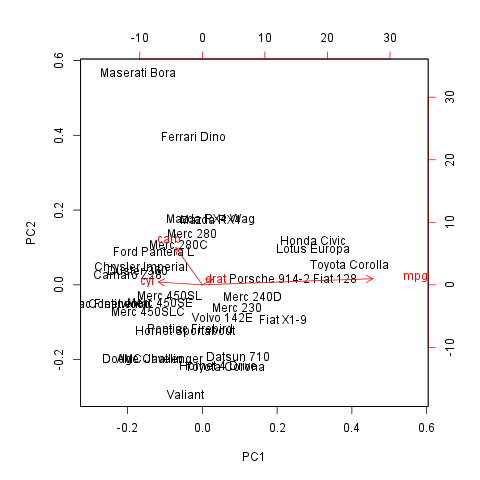## Description

In this template Rapporter will present you Principal Component Analysis.

### Introduction

Principal Component Analysis is a dimension reduction method. It produces linearly independent principal components using the variances of the observations in a set of variables.

### Results

PC1 PC2 PC3
Standard deviation 6.298 1.35 0.9088
Proportion of Variance 0.9354 0.04298 0.01947
Cumulative Proportion 0.9354 0.9783 0.9978

From the table above one can see that the first 3 Principal Components contains the 93.535 %, 4.298 % and 1.947 % of the variances, so together the 99.78 % of that.

##### Visual representation

It could be informative to see visually how the observations lies on these components. On that two dimensional plot below, where the axes are the components which contains the two most variances, you can see (the red vectors) the effect of the variables as well.#### Rotation

As you wanted to check the Rotation matrix let us present that for you:

PC1 PC2 PC3
carb -0.1486 0.9728 -0.08587
mpg 0.9557 0.1614 0.2433
cyl -0.2476 0.07389 0.9502
drat 0.05777 0.1488 -0.1745

The cells written in bold shows which components explain the most variances of the variables, with the help of them we can draw the following conclusion:

• PC1 is a principal component of mpg
• PC2 is a principal component of carb
• PC3 is a principal component of cyl

We can say that none of these impacts are negative.

## Description

In this template Rapporter will present you Principal Component Analysis.

### Introduction

Principal Component Analysis is a dimension reduction method. It produces linearly independent principal components using the variances of the observations in a set of variables.

### Results

PC1 PC2 PC3
Standard deviation 6.298 1.35 0.9088
Proportion of Variance 0.9354 0.04298 0.01947
Cumulative Proportion 0.9354 0.9783 0.9978

From the table above one can see that the first 3 Principal Components contains the 93.535 %, 4.298 % and 1.947 % of the variances, so together the 99.78 % of that.

##### Visual representation

It could be informative to see visually how the observations lies on these components. On that two dimensional plot below, where the axes are the components which contains the two most variances, you can see (the red vectors) the effect of the variables as well.#### Rotation

As you wanted to check the Rotation matrix let us present that for you:

PC1 PC2 PC3
carb -0.1486 0.9728 -0.08587
mpg 0.9557 0.1614 0.2433
cyl -0.2476 0.07389 0.9502
drat 0.05777 0.1488 -0.1745

The cells written in bold shows which components explain the most variances of the variables, with the help of them we can draw the following conclusion:

• PC1 is a principal component of mpg
• PC2 is a principal component of carb
• PC3 is a principal component of cyl

We can say that none of these impacts are negative.

This report was generated with R (3.0.1) and rapport (0.51) in 0.891 sec on x86_64-unknown-linux-gnu platform.